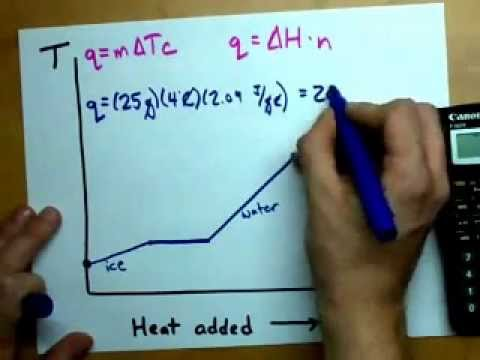# Chemistry Help!!?

2. What energy, in kcal, is needed to change 15.0 g of ice at -10oC to 15.0 g of water at 0 degrees celsius?

3. If 13.4 kJ of energy is added to 1.0 kg of ice at 0CC, how much water is produced? How much ice is left?

4. Using the data below, calculate the amount of energy in kJ required to melt a 16.2 g

aluminum pop can. Assume that the initial temperature of the aluminum is 20oC. Give

 molar heat of fusion of Al (s) = 10.7 KJ/mol

 melting point of aluminum = 660oC

 heat capacity of Al (s) = 0.900 J/goC

Relevance
• 2.

(2.087 J/(g·°C)) x (15.0 g) x (0 - (-10))°C =

313.05 J to warm the ice to its melting point

(333.6 J/g) x (15.0 g) = 5004 J to melt the ice

313.05 J + 5004 J = 5317.05 J = 1.27 kcal

3.

(13.4 x 10^3 J) / (333.6 J/g) = 40.2 g water

(1000 g) - (40.2 g) = 959.8 g ice left

[Adjust the number of significant digits as you see fit.]

4.

(0.900 J/g·°C) x (16.2 g) x (660 - 20)°C = 9331.2 J =

9.3312 kJ to heat the Al to its melting point

(10.7 kJ/mol) x (16.2 g Al / (26.98154 g Al/mol)) = 6.4244 kJ to melt the Al

9.3312 kJ + 6.4244 kJ = 15.8 kJ total

•Log in to reply to the answers
• Anonymous
1 month ago

2. Watch this video and use the formulas in it.  You need to heat the ice to melting, melt the ice and then heat the water to the final temperature.  Ignore the steps on boiling the water and heating the steam.•Log in to reply to the answers